Chess

How many different ways can initiate a game of chess (first pass)?

Result

n =  400

Solution:Leave us a comment of example and its solution (i.e. if it is still somewhat unclear...):Be the first to comment!To solve this example are needed these knowledge from mathematics:

See also our variations calculator. Would you like to compute count of combinations?

Next similar examples:

1. Chess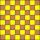How many ways can select 4 fields on classic chess board with 64 fields, so that fields don't has the same color?
2. Toys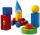3 children pulled 12 different toys from a box. Many ways can be divided toys so that each children had at least one toy?
3. Cars plates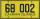How many different licence plates can country have, given that they use 3 letters followed by 3 digits?
4. Olympics metalsIn how many ways can be win six athletes medal positions in the Olympics? Metal color matters.
5. MetalsIn the Hockey World Cup play eight teams, determine how many ways can they win gold, silver and bronze medals.
6. MedalsIn how many ways can be divided gold, silver and bronze medal among 21 contestant?
7. Sweater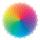Dana confuses sweater and wool has a choice of seven colors. In how many ways she can choose from three colors to the sleeves?
8. Seven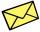Seven friends agree to send everyone a holiday card. How many postcards were sent?
9. PIN - codesHow many five-digit PIN - code can we create using the even numbers?
10. Coin and die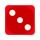Flip a coin and then roll a six-sided die. How many possible combinations are there?
11. 2nd class variationsFrom how many elements you can create 6972 variations of the second class?
12. Pupils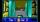Four pupils is going via dark hall. Start at one side and have to get to the other side for 17 minutes. They have only one lamp for the trip. The corridor is narrow, they can therefore go up two pupils at a time and go at the slower speed. Each pupil went.
13. VariationsDetermine the number of items when the count of variations of fourth class without repeating is 42 times larger than the count of variations of third class without repetition.
14. Tricolors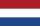From the colors - red, blue, green, black and white, create all possible tricolors.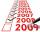Determine the number of integers from 1 to 106 with ending four digits 2006.We throw two dice. What is the probability that max one three falls?We want to prove the sentence: If the natural number n is divisible by six, then n is divisible by three. From what assumption we started?